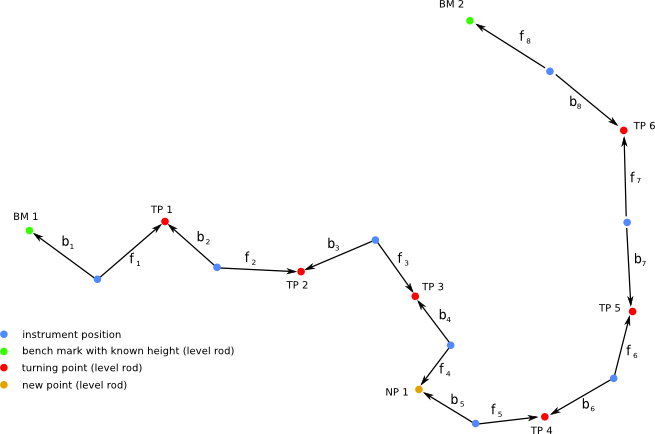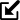# Differential levelling online calculation

 Line and area levelling In Example 2 the determined new point is checked by continuing the measurement to a second benchmark BM 2, the elevation of which is known (of course, alternatively to the first benchmark BM 1 can also be measured back). This also allows the deviation (misclosure) to be calculated. When calculating the adjusted reduced level, the misclosure is distributed evenly over the backsights of the turning points (adjustment). The adjustment is rounded to 3 digits behind the decimal point. If all points have been measured from only one instrument position, no calculation of the adjustment is made (see Example 3). The error evaluation in the second table gives an overview. Also the allowable misclosure may be calculated using the formula constant * √s; s is the length of the levelling section in kilometers (if the length unit meter is selected) or miles (if the length unit foot is selected). The choice of the constant depends on the respective problem and assumes lower values for higher accuracy requirements. Depending on the length unit, the script offers three selectable values for the constant. In Germany, the constant is given a value of 10 or 15 in the literature for construction purposes. For the calculation activate corresponding checkbox and enter the values for the constant and s in the next step. If the resulting allowable misclosure is exceeded, the corresponding value is highlighted in red, i.e. the measurement must be repeated. If the value falls below this value, it is highlighted in green.Example 2